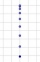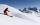Traffic collision

When investigating a traffic accident, it was found that the driver stopped the vehicle immediately after the accident by constant braking on a 150 m track in 15 seconds. Do you approve that the driver exceeded the permitted speed (50 km/h) in the village before braking?

Result

v =  72 km/h

Solution:Leave us a comment of this math problem and its solution (i.e. if it is still somewhat unclear...):Be the first to comment!To solve this verbal math problem are needed these knowledge from mathematics:

Do you want to convert length units?

Next similar math problems:

1. G forcesCalculate deceleration of car (as multiple of gravitational acceleration g = 9.81 m/s2) which occurs when a car in a frontal collision slows down uniformly from a speed 111 km/h to 0 km/h in 1.2 meters trajectory.
2. CollisionThe two bodies, whose initial distance is 240 m, move evenly against each other consistently. The first body has an initial velocity of 4 m/s and an acceleration of 3 m/s2, the second body has an initial speed of 6 m/s and an acceleration of 2 m/s2. Find
3. DriverThe driver of the car at a speed of 100 km/h faced the obstacle and began to brake with a slowing of 5 m/s². What is the path to stopping the car when the driver has registered the obstacle with a delay of 0.7 s?
4. Car crashOn the road, with a maximum permitted speed of 60 km/h, there was a car crash. From the length of the vehicle's braking distance, which was 40 m, the police investigated whether the driver did not exceed that speed. What is the conclusion of the police, as
5. Train 2The train slowed down from 90 km/h to 72 km/h in 5 seconds. How long track travel?
6. Aircraft nose downHow long will fall airliner from a height of 10000 m at speed 1,000 km/h?
7. Rocket startThe body launched vertically up returns to the start site in 6 seconds. What height did it have?
8. WavelengthCalculate the wavelength of the tone frequency 11 kHz if the sound travels at speeds of 343 m/s.
9. Free fallThe free fall body has gone 10m in the last 0.5s. Find the body speed at the moment of impact.
10. Theorem proveWe want to prove the sentence: If the natural number n is divisible by six, then n is divisible by three. From what assumption we started?
11. Pre účinnosťPre účinnosť bŕzd osobného vozidla je predpísané, že automobil pohybujúci sa po vodorovnej vozovke rýchlosťou 40km. H musí zastaviť na dráhe 15,4 m. Aké veľké je pritom spomalenie automobilu?
12. BomberThe aircraft flies at an altitude of 4100 m above the ground at speed 777 km/h. At what horizontal distance from the point B should be release any body from the aircraft body to fall into point B? (g = 9.81 m/s2)
13. GravitationFrom the top of the 80m high tower, the body is thrown horizontally with an initial speed of 15 m/s. At what time and at what distance from the foot of the tower does the body hit the horizontal surface of the Earth? (use g = 10 ms-2)
14. BrakesThe braking efficiency of a passenger car is required to stop at 12.5 m at an initial speed of 40 km/h. What is the acceleration braking by brakes?What overload in g (g-force) has passed the pilot if he accelerated from 0 to 600 km/h in 3 seconds?The car accelerates at rate 0.5m/s2. How long travels 400 meters and what will be its speed?At this point, the first skier lead 20 km before the second skier and travels at a constant speed 19 km/h. The second skier rides at 24 km/h. How long take him to catch up the first?